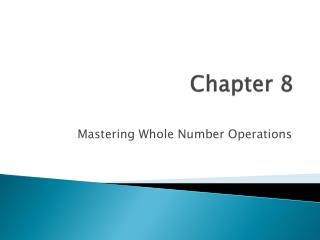Download PresentationChapter 8

# Chapter 8 - PowerPoint PPT PresentationDownload Presentation## Chapter 8

- - - - - - - - - - - - - - - - - - - - - - - - - - - E N D - - - - - - - - - - - - - - - - - - - - - - - - - - -
##### Presentation Transcript

1. Chapter 8 Mastering Whole Number Operations

2. Basic Facts • Addition and Multiplication involves all single digit addends and factors • Addend plus addend equals sum • Factor times factor equals product • How many basic facts are there????

3. Basic Facts • 100!!!! For each addition and multiplication • How???

4. Three Step Approach to Fact Mastery 1. Understand the meaning of the operations 2. Use thinking Strategies to Retrieve Facts *use facts that they know to figure out facts that they don’t i.e. 2+2 =4 so 2+3 must be one more than that * other stategies include counting on, counting back, skip counting, one more, one less…

5. Three step approach cont… 3. Using Consolidating Activities for Drill and Practice games , activities , worksheets all help children memorize these facts

6. Thinking Strategies for Addition and Subtraction • Counting on … 6+3 start from the 6 and count on three times seven, eight , nine • Remind children to start from the larger # regardless of order of the problem ..3+6 still starting at 6 and add on three more • This method works well for both addition and subtraction • Sometimes a calendar works as a good visual to use this method—see next slide

7. One more or one less • Use counters • Show two groups of 6 • “How much is 6+6?” • Add one more to one of the groups • Now we have 6 + 7. “How many altogether?” • One more than twelve • Count out 13 objects with students • 6+6 = 12 then 6 + 7 = 13

8. Compensation • One addend is increased while the other addend is decreased by trying to make one addend 10 • Use counters to model two piles 8 + 4=? • Manipulate counters so one pile has 10 • Now solve

9. Commutative Property of Addition and Multiplication • Order doesn’t matter • 3+5 = 5+3 3•5 = 5•3 However this does not work for Subtraction and Division 6-4 ≠ 4-6 24/6 ≠6/24

10. Calculator Game • 4 • 7 • 17 • 37 • 33 • 13 • 53 • 59 • 89

11. Calculator/ broken calculator • John’s calculator is broken. The number 4 key will not work. How may possible combinations can you come up with on the screen to get 4 without actually using 4? • John has a math problem for hw and he is still trying to use the same calculator to solve this problem 24-4= ? Can you help him rewrite this problem so he can use his calculator?